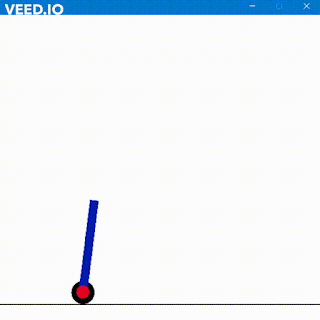## PARITY BIT CHECKER

Hola Amigos
Here is the code for the parity bit checker in System Verilog
The output will be shown in the transcript.
I have used ModelSim sometimes using Xilinx iSim as second.

Code
module func();
reg [7:0] data;
reg  parity;
integer i;

function abc;
input [31:0] data;
integer i;
begin
abc = 0;
for (i= 0; i < 32; i = i + 1) begin
abc = abc^data[i];   //EXOR
end
end
endfunction

initial begin
parity = 0;
data = 0;
for (i=145; i<160; i = i + 1) begin
#5 data = i; // FOR different data values
parity = abc(data);
\$display ("DATA = %b, Parity-bit = %b", data, parity);
end
#25 \$finish;
end

endmodule

Output